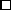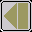### Theorem B

The function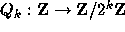defined by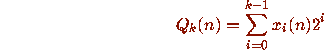is periodic with period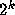. The induced function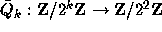is a permutation, and its order is a power of 2.

### Proof B

(sketch). The theorem is established by induction on k, using the inductive hypotheses:

(1)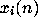is periodic with period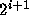for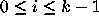. In fact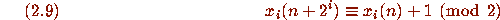for.

(2)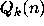is periodic with period.

(4)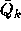is a permutation whose order divides. Also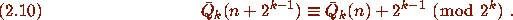I omit the details.